# Quantum Computing with IBM Q

## What you need

• Any computer with a Web browser.

## Purpose

To practice using a quantum computer for very basic operations.

## Background

Here is a quick introduction to quantum mechanics and quantum computing. Click any of the three images below for a web page explaining each topic in more detail.

### The Feynman Double Slit Experiment

Quantum mechanical objects, such as electrons, are not localized when they are not observed. They move as waves, smeared across space, but when observed, the wave function collapses and they are only seen to be at one location. Here is a famous explanation of that phenomenon.### Shor's algorithm

This can be used to construct a network of qubits that factors compound numbers into their prime factors very quickly, as explained here: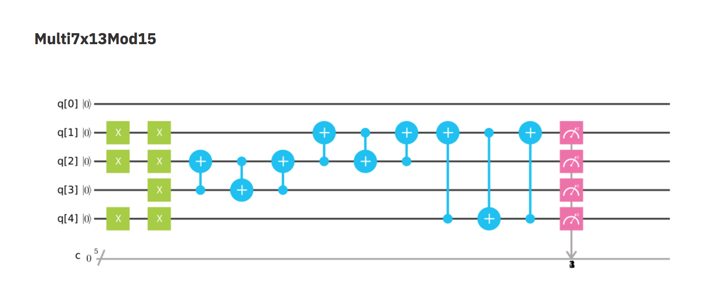### Qubits

In Quantum Computing, the fundamental element is the Qubit, an object that has many possible states, as shown below.

At the top is the pure state `|0>` and at the bottom is the pure state `|1>`. Normal computers use bits with only these two states: 0 and 1.

Quantum computers use the whole range of states, including the intermediate states that are "superpositions" of `|0>` and `|1>`.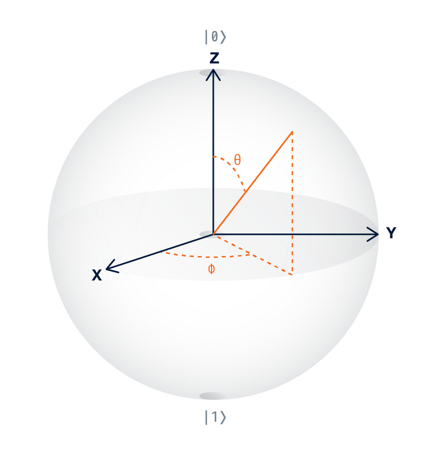## Getting Started

This project gives you a little practice with the fundamental operations used in quantum computing.

In a Web browser, go to

Click the "Start experimenting with a quantum computer" button, as shown below.The next page shows the "Composer". Here we'll program a quantum computer, using the chart at the bottom with five lines, like a music composer's score.

At the top is the Internals section, showing the details of IBM's actual quantum computer. Ignore it for this project.

At the lower left is the Score where you will create your programs, by dragging Gates from the right and dropping them.

The pink icon with a white umbrella-looking figure on it is called a "Measurement Gate". Drag a measurement gate onto the score and drop it there, as indicated by the pink arrow in the figure below.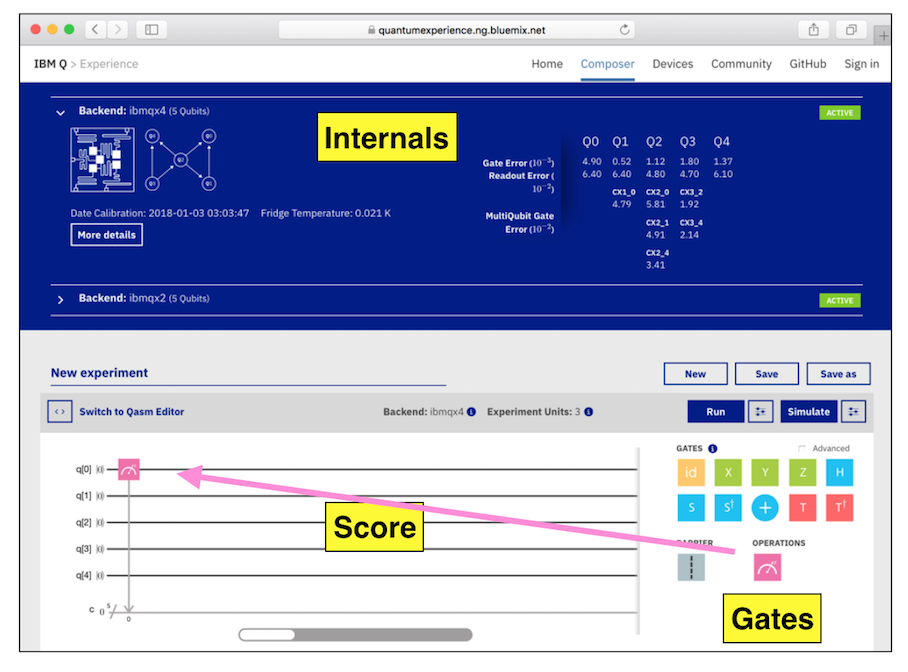At the center right, click the Simulate button.It puts you on a forum page. Refresh the page to implement the log in. Then, from the top menu, click Learn, Composer, as shown below.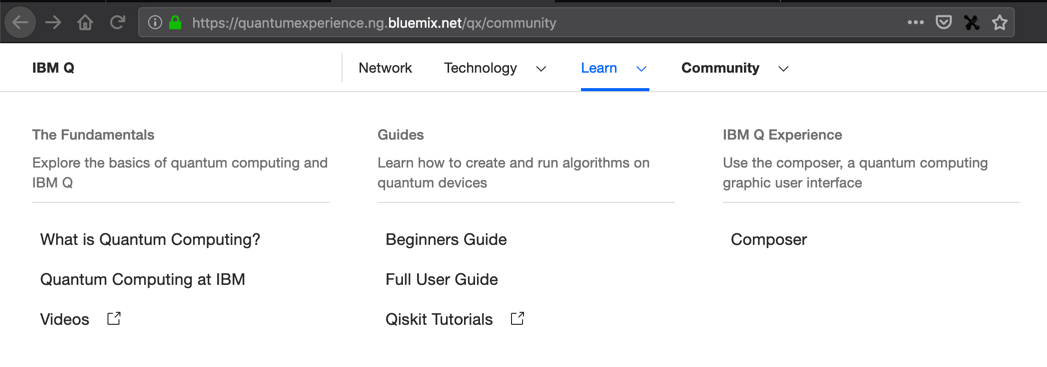Drag the pink "Measurement Gate" onto the score and drop it there again.

Click the Simulate button.

A box pops up asking you to "Name your experiment". Click OK.

"Your Quantum Results" appear, including the "Quantum State: Computation Basis" chart shown below.

This chart shows the results of running your experiment 100 times. Every time, the result was the same: 00000, as shown at the bottom of the purple bar.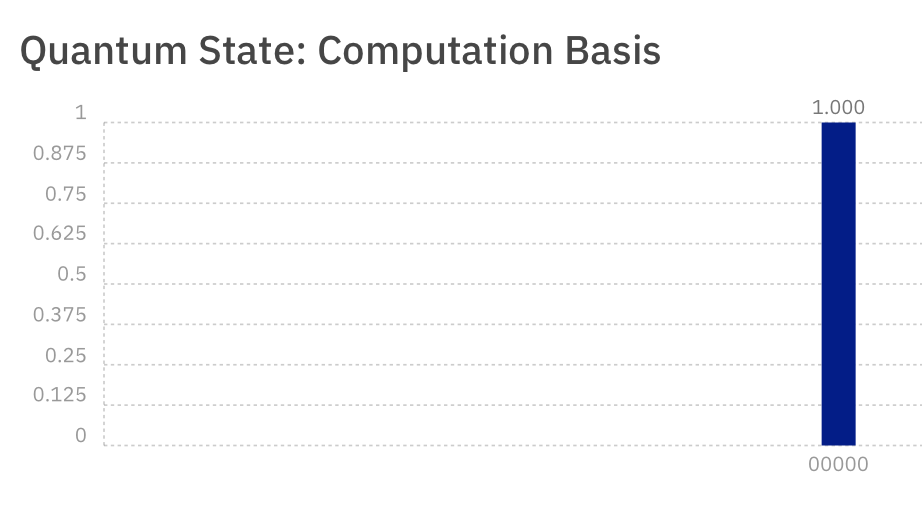## Classical Measurement

The experiment you just created is shown below.

It did not demonstrate any quantum behavior. There are five Qubits, and they all start in the "Zero" state, written as

``` |0> ```
All you did was measure one Qubit, and the result was always 00000 because all the Qubits are stable in their zero state.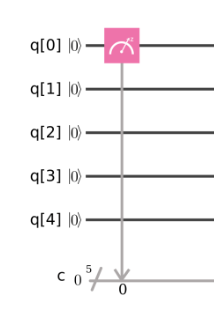## More Classical Measurements

Close the "Your Quantum Results" box. Drag more "Measurement Gates" onto the score, as shown in the lower portion of the image below.

Click the Simulate button. You get the same result: 00000 every time, as shown in the upper part of the image below.

This is how ordinary non-quantum processors work. Measuring a bit has no effect on it--you can measure it anytime you want.## Flipping a Bit

Close the "Your Quantum Results" box.

Drag gates as needed to make the quantum score shown below, and Simulate it.

The green X gate flips a Qubit from `|0>` to `|1>`

This is still the same as a normal processor: no quantum effects are visible.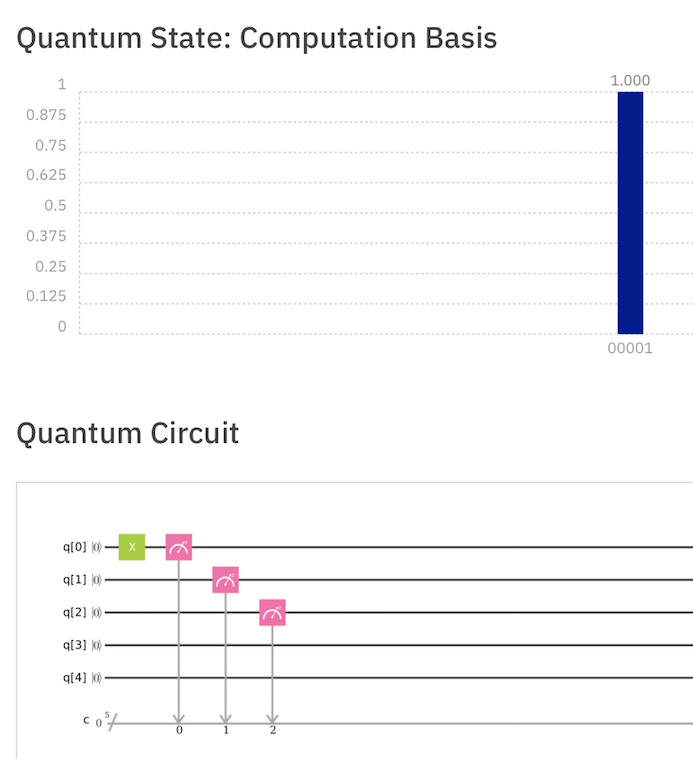## Creating Uncertainty ("Superposition")

Close the "Your Quantum Results" box.

Drag gates as needed to make the quantum score shown below, and Simulate it.

The blue H gate is called a "Hadamard" gate, and it changes a Qubit from its `|0>` state to a "Superposition" state, mixing equal portions of the `|0>` state and the `|1>` state.

This is also called "Uncertainty" and it's the bizarre part of quantum mechanics: quantum objects in a "Superposition" state are neither 1 nor 0, but in an uncertain state with a probability of either result.

So the measurement doesn't always give the same result. Half the time the result is 00001 and the other half of the time it's 00000.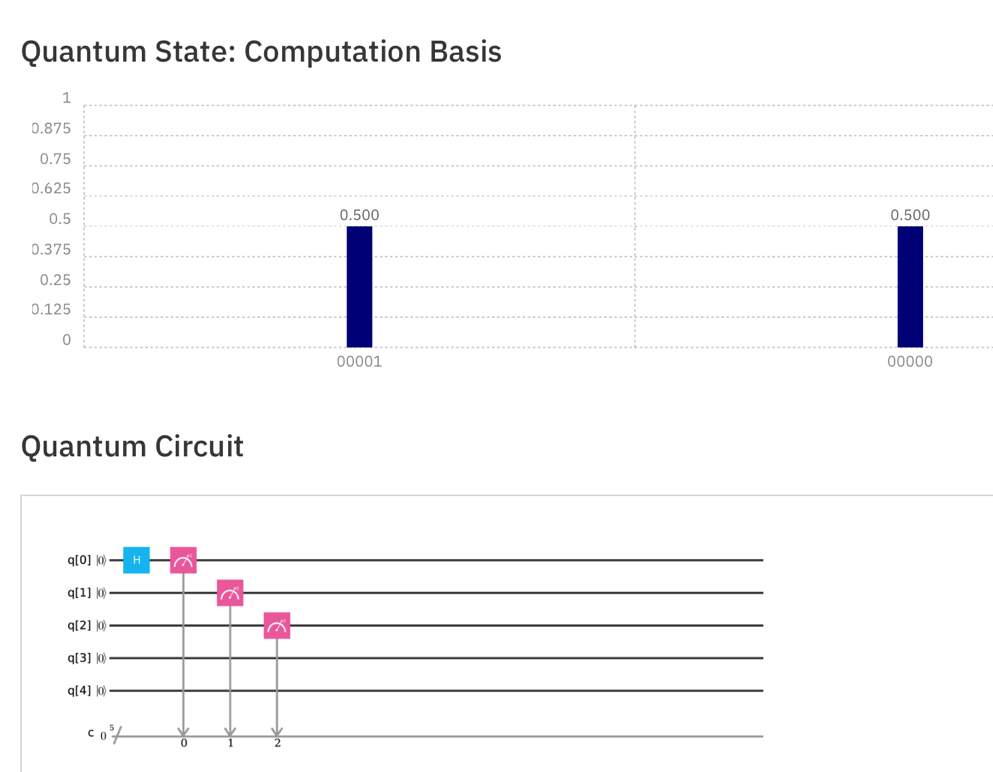## Three Qubits in Superposition States

Close the "Your Quantum Results" box.

Drag gates as needed to make the quantum score shown below, and Simulate it.

The three Qubits are all in Superposition states, so there are eight possible results with equal probability.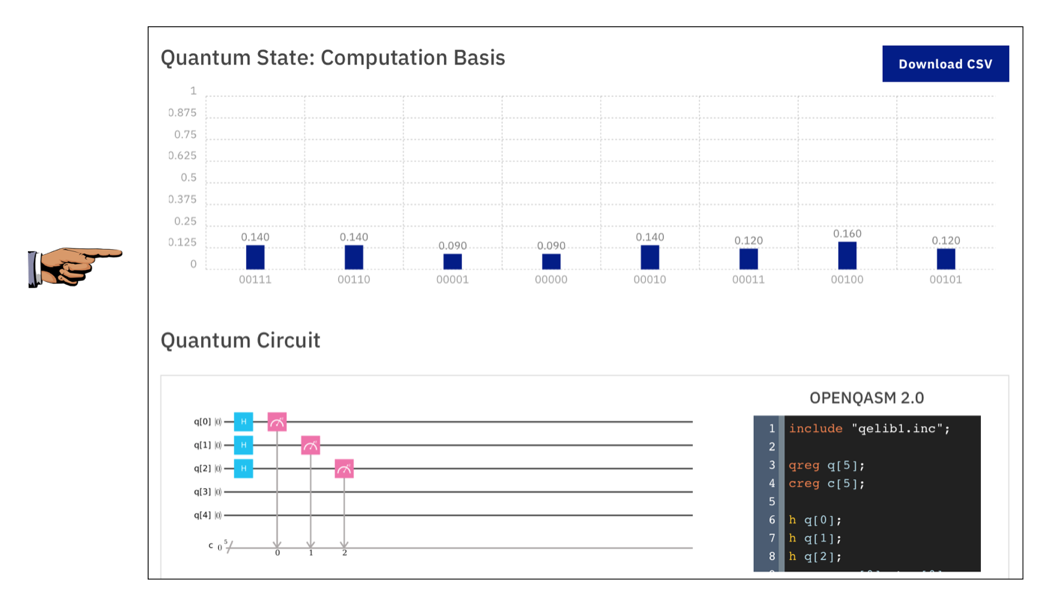## One Uncertain Qubit

Close the "Your Quantum Results" box.

Drag gates as needed to make the quantum score shown below, and Simulate it.

The H gate makes the second Qubit uncertain, but the other two Qubits are stable in the `|0>` state,.

The only two possible answers are 00000 and 00010, as shown below.## Entanglement

Close the "Your Quantum Results" box.

Drag gates as needed to make the quantum score shown below, and Simulate it.

The + gate Entangles two Qubits, so they will always give the same result when measured. So Qubit 0 now follows Qubit 1, and the possible results are now 00000 and 00011, as shown below.## Entanglement with Bit-Flip

Close the "Your Quantum Results" box.

Drag gates as needed to make the quantum score shown below, and Simulate it.

Now Qubits 0 and 1 are entangled, but always opposite, so the possible results are 00001 and 00010, as shown below.# Challenge 1: Run This Program (10)

Build and simulate the program shown below. Combine all the bits of the possible answers and use the form below to put your name on the WINNERS PAGE.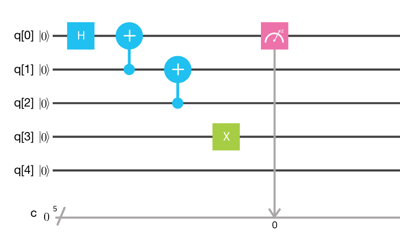Your Name (without spaces): All the answer bits like this: ``` 0000011111```

## Challenge 2: Four or Five Entangled Qubits (No points)

Entangle four Qubits, so there are only two possible answers: 00001 and 10111, as shown below.

One of my students succeeded in entangling all five Qubits.

This is interesting, but it's not worth any points in Crypto Hero because I couldn't figure out anything to submit to prove you completed it.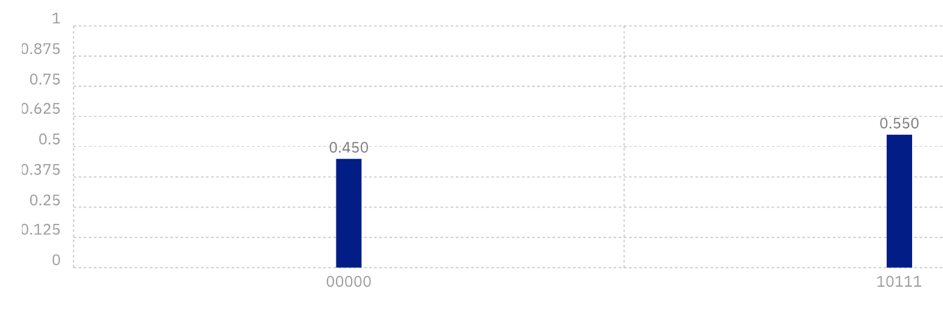## Sources

IBM Q Beginner's Guide

Posted 1-3-18 by Sam Bowne
Added to Crypto Hero 4-16-18 8:45 am
Image added to make challenge possible 6-2-18